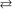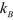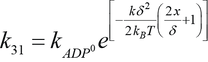Duke 1999 three state model

Following the approach of Duke (1999, 2000), we formulated forward and backward state transition rates of the chemical reactions for the simplified three state cycle, for convenience denoted by both numeric and generic state labels: 1 = M.ADP.Pi, 2 = A.M.ADP.Pi, 3 = A.M.ADP. These transition rates are consistent with Kramers' theory and completely specify the strain dependence of transition rates between these states.

1. For binding M.ADP.Pi     A.M.ADP.Pi the activation energy is supplied by thermal (Brownian) fluctuations. The elastic element of the detached myosin molecule fluctuates thermally and the strain-dependent binding rate varies with displacement between associated actin and myosin site,where       is overall binding rate of M·ADP·Pi to actin,    is crossbridge stiffness,     is absolute temperature and       is Boltzmann’s constant. The reverse reaction occurs at a constant rate                  .

2. Two states A·M·ADP·Pi and A·M·ADP are assumed to be in dynamic equilibrium if [Pi]>0 because the forward/backward reaction between them is rapid. Also the Pi release is accompanied by a large (negative) change in chemical free energy            . Thus, to define the population of these states it suffices to specify the ratio of forward and reverse rates (i.e., ratio of the relative probabilities of these post- and states pre-power stroke) that also includes the change in elastic energy of the power stroke:where,     is displacement of the lever arm after carrying out the power stroke.

3. For strain dependent ADP release (i.e., A.M.ADP      A.M), the difference between initial and final strain energies gives the forward rate as:where      is displacement that lever arm must move to open the nucleotide pocket and allow ADP release, and           is the rate of ADP release when the elastic element is relaxed. ATP binding is much faster than the reverse rate        , and thus any strain dependence due to conformational change has almost no effect. It is therefore reasonable to take     to be small and constant. Furthermore, ATP-mediated detachment is sufficiently faster than the reverse reaction that the latter can be safely neglected.